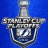# Mobius (Demarks Pivot and Demarks MA) for ThinkorSwim#### mc01439

##### Well-known member
2019 Donor
VIP
Mobius posted these on "thinkScript Lounge" this morning.

10:17 Mobius: Here are two studies by DeMark I find still useful.
This one I find useful as boundaries for intraday likely moves:

https://tos.mx/pGukThm
https://tos.mx/vBx2ibC
Code:
``````#Start Code
# Demark's Pivots
# Ported to TOS by Mobius
#

declare hide_on_daily;

def o = open(period = AggregationPeriod.Day);

def h = high(period = AggregationPeriod.Day);

def l = low(period = AggregationPeriod.Day);

def c = close(period = AggregationPeriod.Day);

def x = if c < o

then h + (2 * l) + c

else if c > o

then (2 * h) + l + c

else if c == o

then h + l + (2 * c)

else x;

plot Pivot_Point = X/4;

Pivot_Point.SetPaintingStrategy(PaintingStrategy.Horizontal);

plot Support = X/2 - h;

Support.SetPaintingStrategy(PaintingStrategy.Horizontal);

plot Resistance = X/2 - l;

Resistance.SetPaintingStrategy(PaintingStrategy.Horizontal);

# End Code``````

This is still a very good Moving Average

Code:
``````#Start Code

# Demarks MA

# Mobius

# V01.2.2011

# What makes this MA unique is that it can be run at very low period lengths and still be a smooth indicator. Which makes it very responsive.

script Range{

input d = close;

input Min = 0;

input Max = 10000;

def hh = HighestAll(d);

def ll = LowestAll(d);

plot nr = (((Max - Min) * (d - ll)) /

(hh - ll)) + Min;

}

input n = 5;

input V = 0.682;

def newMax = Highest(high, n);

def newMin = Lowest(low, n);

script BP {

def bp = (((close - open) / (high - low)) * volume);

plot data = bp;

}

def newBP = Range(BP().data, newMin, newMax);

def StDev = StDev(newBP, n);

def GDEMA = (ExpAverage(newBP, n)* (1 + V)) -

(ExpAverage((ExpAverage(newBP, n)), n)* V);

plot PlotData = if IsNaN(newBP)

then Inertia(GDEMA, n)

else Inertia(GDEMA, n);

PlotData.SetStyle(Curve.Firm);

PlotData.SetLineWeight(1);

plotData.AssignValueColor(if PlotData > PlotData

then Color.Green

else Color.Red);

# End Code``````

10:18 Mobius: There 2 different studies in the post above.

Last edited by a moderator:
•markos, skynetgen and BenTen#### markos

##### Well-known member
VIP
@mc01439 Thanks for grabbing these gems. Mobius said he was on 2 minute charts for /ES.
What are the Ticks you used today for the pic above?#### korygill

##### Active member
VIP
Interesting indicators, thx for sharing @mc01439. Looks like you have created some cool studies and ways to track signals with those labels too. Are any of those already on this site?#### korygill

##### Active member
VIP
Appreciate your sharing @mc01439. Always looking to learn new things and incorporate into my own work.

H

#### HighBredCloud

##### Well-known member
VIP
about the DeMarks MA...just an observation but isn't a HULL MA smoother?Fractal Pivot Strategy Mobile by Mobius Questions 11